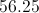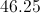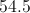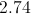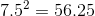# High School Math : Understanding Variance

## Example Questions

### Example Question #37 : Basic Statistics

A bird watcher observed how many birds came to her bird feeder for four days.  These were the results:

Day 1: 15

Day 2: 12

Day 3: 10

Day 4: 13

What is the variance of the number of birds that visited the bird feeder over the four days?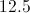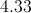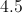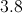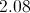Explanation:

Variation measures the average difference between values within a group.  The process is not complicated but there are four steps that can take time.  First, find the mean of the values.  Second, subtract the mean from the first value and square the result.  Do this for all remaining values.  Third, add these results together.  Fourth, divide this value by the number of values in the group minus one (in this case, there are four days).

1: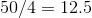2: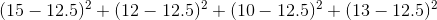3: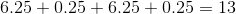4: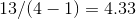Note that to find the standard deviation, we would simply take one additional step of finding the square root of the variance.

### Example Question #1721 : High School Math

Alice recorded the outside temperature at noon each day for one week. These were the results.

Monday: 78

Tuesday: 85

Wednesday: 82

Thursday: 84

Friday: 82

Saturday: 79

Sunday: 80

What is the variance of the temperatures?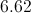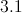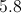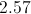Explanation:

There are four steps to finding the variance of values within a group.  First, find the mean of the values.  Second, subtract the mean from the first value and square the result.  Do this for all remaining values.  Third, add these results together.  Fourth, divide the result  by the number of values in the group minus one (in this case, there are seven days, so you must divide by six).

### Example Question #1 : How To Find The Standard Deviation For A Set Of Data

The standard deviation of a population is 7.5.  What is the variance of the population?